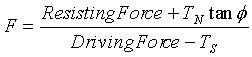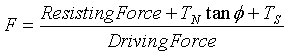Join one of our upcoming courses taking place around the world! Explore now

# How Bolts are Implemented

Bolts are implemented in a RocPlane planar wedge stability analysis as follows:

## Capacity and Orientation

• Bolts affect the Factor of Safety through their Capacity and Orientation (Angle).
• Bolt Capacities and Orientations are added vectorially and are included in the Factor of Safety calculation as a single, equivalent force passing through the centroid of the wedge. Either the Active or Passive Bolt Model applies (see below).
• Multiple bolts that have the same Orientation can therefore be simulated by a single bolt having the same total Capacity.

## Length and Location

• If the Anchored Length of a bolt = 0 (i.e., if it does not pass through the wedge), the bolt has NO effect on the model - its effective Capacity is zero.
• The Location of bolts (on the face of the wedge) has some effect on the Factor of Safety. Even though all forces in the wedge stability analysis are assumed to pass through the centroid of the wedge , the exact location of a bolt can affect the anchor length of the bolt. Where a selected bolt property type utilizes anchor length for its bolt capacity calculations, the Factor of Safety can be affected.

## Bolts vs. External Force

• If the Bolt Model is Active, a bolt is exactly equivalent to an External Force with the same magnitude and orientation.

TIP: As a suggested exercise, you can verify that a bolt using the Active Bolt Model or an equivalent External Force results in the same Factor of Safety for a given wedge.

• If the Bolt Model is Passive, a bolt and an External Force with the same magnitude and orientation are equivalent,

## Active and Passive Bolt Models

In general terms, the Factor of Safety is defined as the ratio of the forces resisting motion to the driving forces. Driving forces include the mass of the wedge accelerated by gravity, seismic forces, and water pressure. Resisting forces arise from the cohesion and frictional strength of the sliding plane (Failure or Discontinuity Plane).

### ACTIVE SUPPORT

Active support is included in the RocPlane analysis as shown in Equation 1 below:Eqn. 1

Active Support is assumed to act in such a manner as to DECREASE the DRIVING FORCE in the Factor of Safety calculation. Tensioned cables or rockbolts, which exert a force on the wedge before any movement has taken place, are considered Active support.

NOTE: External Forces are incorporated in the RocPlane analysis in the same manner as Active support (i.e. according to Equation 1).

### PASSIVE SUPPORT

Passive Support is included in the RocPlane analysis as shown in Equation. 2.Eqn. 2

By this definition, Passive support is assumed to INCREASE the RESISTING FORCE provided by shear restraint in the Factor of Safety equation. Untensioned dowels or grouted cable bolts, which only develop a resisting force after some movement of the wedge has taken place, are considered Passive support.

Since the exact sequence of loading and movement in a rock slope is never known in advance, the choice of Active or Passive Bolt Models is somewhat arbitrary. The user may decide which of the two models is more appropriate for the wedge being analyzed. In general, Passive support always gives a lower Factor of Safety than Active support.